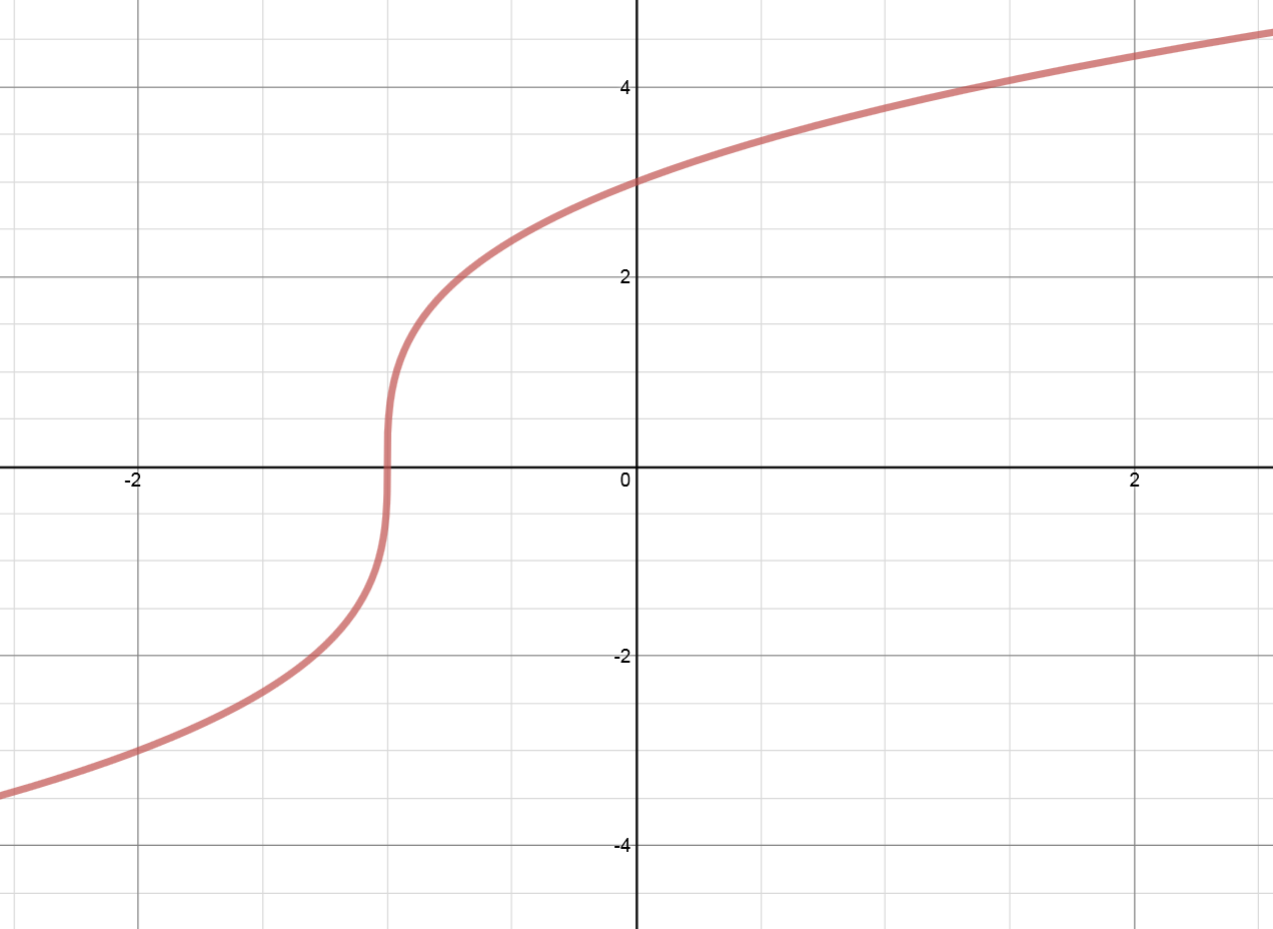# Transformations of functions: Vertical stretches

### Transformations of functions: Vertical stretches

#### Lessons

• Introduction
An Experiment to Study "Vertical Stretches"
Sketch and compare: $\left( y \right) = {x^2} + 2$
VS.
$\left( {2y} \right) = {x^2} + 2$
VS.
$\left( {\frac{y}{3}} \right) = {x^2} + 2$
a)
a) Sketch all three quadratic functions on the same set of coordinate axes.

a)
Compared to the graph of $\left( y \right) = {x^2} + 2$:
$\left( {2y} \right) = {x^2} + 2$ is a vertical stretch about the x-axis by a factor of ____________.
$\left( {\frac{y}{3}} \right) = {x^2} + 2$ is a vertical stretch about the x-axis by a factor of ____________.

• 1.
Vertical Stretches
Given the graph of $y = f\left( x \right)$ as shown, sketch:
a)
$y = \frac{1}{2}f\left( x \right)$

b)
$y = \frac{4}{3}f\left( x \right)$

c)
In conclusion:
$\left( y \right) \to \left( {2y} \right)$: vertical stretch by a factor of ________ ⇒ all $y$ coordinates ______________________.
$\left( y \right) \to \left( {\frac{3}{4}y} \right)$: vertical stretch by a factor of ________ ⇒ all $y$ coordinates ______________________.